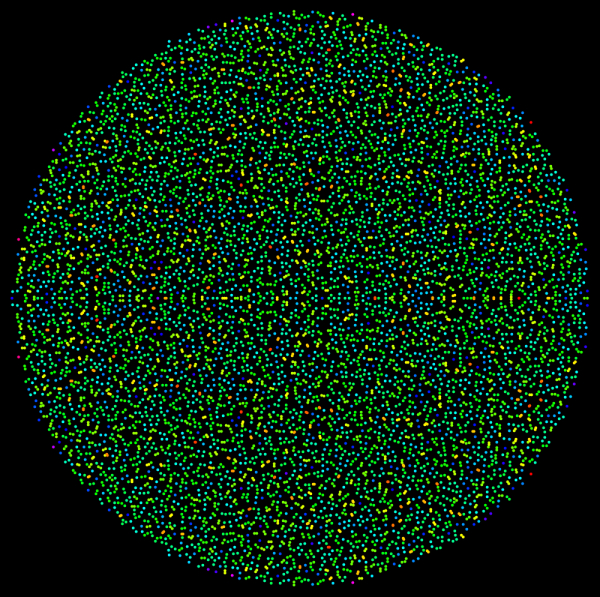M A T H 2 1 B
Mathematics Math21b Spring 2008
Linear Algebra and Differential Equations
Exhibit: Girkos Circular Law
 The eigenvalues of a random 8000x8000 matrix colored according how close the nearest neighboring eigenvalue is. The plot was generated with the following Mathematica lines: ```M=8000; A=Table[Random[]-1/2,{M},{M}]; e=Eigenvalues[A]; d=Table[Min[Table[If[i==j,10,Abs[e[[i]]-e[[j]]]],{j,M}]],{i,M}];a=Max[d]; b=Min[d]; Graphics[Table[{Hue[(d[[j]]-a)/(b-a)],Point[{Re[e[[j]]],Im[e[[j]]]}]},{j,M}]] ``` It illustrates Girko's circular law in the fancy area of random matrix theory. You explore this a bit in the Mathematica lab. Try it out with smaller M, the above case needs a while to complete. Girkos law applies when the entries of the matrix are Gaussian random variables but it is quite robust. Results on limiting distributions are versions of central limit theorems.